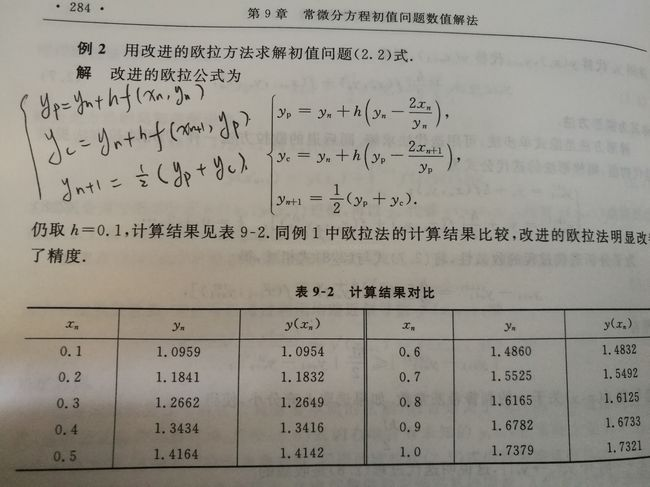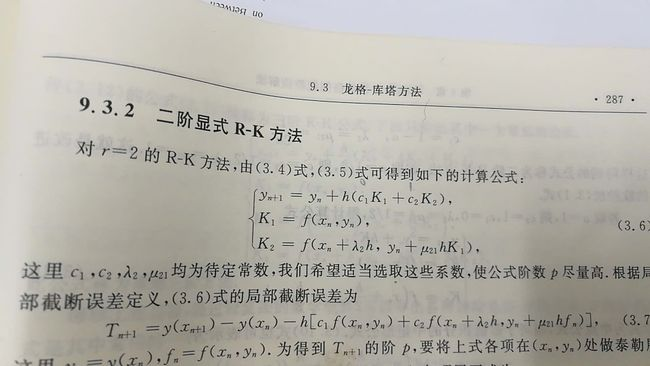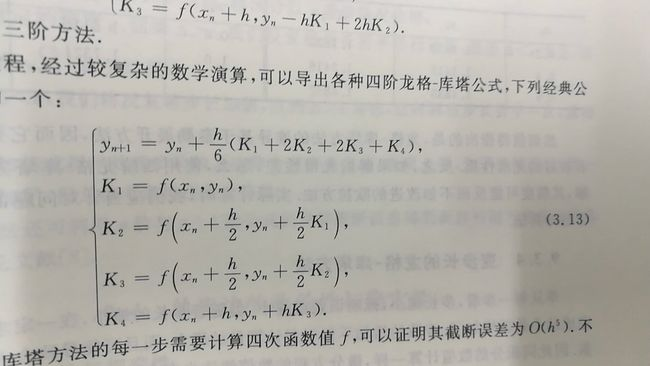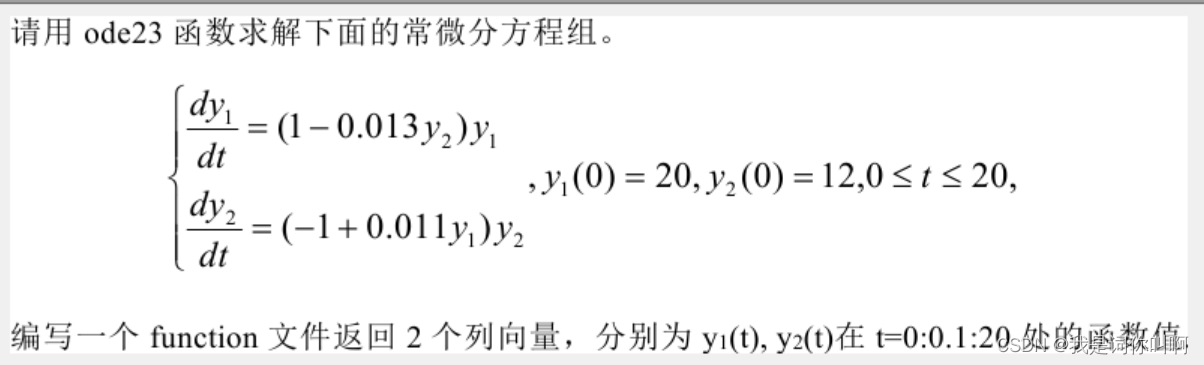• ode23
2021-04-22 05:57:21

我正在尝试将我的代码从MATLAB翻译成Python。我遇到的问题是如何将一个ODE翻译成Python。我在网上读到了我可以用的东西scipy.integrate.ode/scipy.集成.odeint为了这个。不幸的是，我不能得到我想要的方式。在

我的MATLAB代码如下：function [W] = PSFFA_MM1 (arrivalRate, serviceRate)

tspan = 1:1:96;

ic = 0;

opts = odeset('RelTol',1e-2,'AbsTol',1e-4);

[t,x] = ode23(@(t,x) myode(t,x,arrivalRate, serviceRate), tspan,ic,opts);

function dxdt = myode(t,x,lambda, MU)

l = interp1(lambda, t);

mu = interp1(MU, t);

dxdt = -mu*(x/(x+1)) +l;

end

W = x./arrivalRate;

end

我的Python代码如下：

^{pr2}\$

我正在努力解决的一个问题是，在MATLAB中不需要显式地指定'x'，而这必须在Python中明确指定。在

我知道这不是一个具体的问题，但我希望有人能进一步帮助我。在

更多相关内容
• 今天说一说MATLAB中ode23函数的原理，在网上看了好多，但是不知道是怎么计算的，就知道是那么用的，但是最后结果咋回事不知道，今天来讲一讲是怎么计算的。首先来个程序：function f=eg6fun(t,y)f=-y^3-2;end上面是...

今天说一说MATLAB中ode23函数的原理，在网上看了好多，但是不知道是怎么计算的，就知道是那么用的，但是最后结果咋回事不知道，今天来讲一讲是怎么计算的。

首先来个程序：

function f=eg6fun(t,y)

f=-y^3-2;

end上面是我定义的一个函数，看着挺简单的哈！不多说了。

[t,y]=ode23(@eg6fun,[0,30],1);这句话是我用ode23调用的语句，先说一下，这里eg6fun是我上面函数的名称，也就是ode23主要计算的就是这个函数的微分。[0,30]为t的范围，这里t没有什么太大的作用，只是为了计算步长用的，之后我把运行后的t和y数据粘在这里，我们发现，在MATLAB中步长并不是固定的。这里应该是用一个什么函数求得，我没查，感兴趣的自己查一下。1为y的初值，也就是我们常常说的y0。

先粘上实验结果，我们在分析怎么来的：

下面的是t的值，这里MATLAB将t在[0,30]区间分成了67份，我这里只粘了一部分：

0

0.0266666666666667

0.0974376132058027

0.178185989598692

0.270160382755732

下面是y的结果，y最后也是一个[1,67]的矩阵：

1

0.922959859735161

0.740501273051361

0.556672216994644

0.363414446549133

下面我们来说是怎么计算的吧！看下面的图，这个是我在数值分析书上照的，其实ode23就是龙格库塔函数的应用，而龙格库塔函数就是根据欧拉法得来的，看下图：上面图片中有三个公式，第一个公式h后面括号中的内容就是要求积分的函数，就是我们程序中的eg6fun。那么就好办了，把图中公式中的括号中的内容换成我们的公式也就是

-y^3-2

然后计算就好了。这里h为步长，也就是我们程序中t的步长，我们可以看到第一次t为0.0266666666666667，而下一次的步长为0.0974376132058027-0.0266666666666667，只要这么一步一步计算就好了。

(这里看图中黑色笔手写的公式)

这里计算一步来表示计算的大概过程：

例如： (1)计算Yp=y1+h * (-y1^3 - 2) = 1 - 0.027*3 = 0.919

(2)       Yc=y1+h * (-Yp^3 - 2) = 1 - 0.027*(0.919^3 - 2) = 0.925

(3)        Y(n+1) = 1/2 * (Yp + Yc) = 1/2 * (0.919 + 0.925) = 0.922

因为这里我们保留精度为3位小数，可能计算的有些误差。还有一点需要注意，龙格库塔函数是对欧拉方法进行的改进，其实龙格库塔函数的精度要比欧拉方法更高。因此这里计算有些许误差。但是大概的过程就是这样的。

上面的内容是之前写的，讲解的是欧拉算法计算微分的过程，其实龙格-库塔方法后来在书中看到，下面介绍一下龙格库塔方法：MATLAB中的ode23就是用的二阶的龙格库塔方法，就是图中3.6的三个公式，这里h为步长，上面给出的t，c1和c2是系数，这个系数取值不是固定的，MATLAB中是啥我也不是确定，但是书中最后给的是c1=0，c2=1，λ2和μ21取值1/2。这样一来，计算一波：y1=1;求y2，将y1带入公式中的yn，这里没有x，所以有x的项可以忽略

k1=-3；

k2=f(1-(1/2)*0.0267*3)=f(0.96)=-2.88

y2=1-0.0267*2.88=0.923

y2求出，其余的过程都是这样求得。ode45是四阶龙格库塔函数，下图为4阶求法，这里不再做介绍：到此MATLAB中ode23的计算方法已经讲解完了，当然，ode45跟这个应该类似，就是ode45比ode23更精确一点，在MATLAB中，如果我们用ode45会发现，t在[0,30]间分成了167份，很明显精度提高了。其实MATLAB中有好多的函数都是用到了数值分析中的内容，而数值分析就是用我们的笨方法来计算数值的一种工具，这是我自己定义的哈，通过减少误差来使计算出来的数据更准确。

展开全文• 基于BS23算法，已知初始斜率和步长，在误差允许范围内近似所需的准确值
• 类型 问题类型 精度 使用范围 ode45 Nonstiff(非刚性) 中等 大多数时候。这应该是你尝试的第一 ...

类型             问题类型                               精度                         使用范围

ode45          Nonstiff(非刚性)                     中等                     大多数时候。这应该是你尝试的第一                                                                                                   个解算器。

ode23          Nonstiff(非刚性)                      低                        用于粗糙误差容许问题或解决中等刚                                                                                                 性问题。

ode113        Nonstiff(非刚性)                     由低到高              用于有严格误差容许的问题或用于解                                                                                                   决计算密集的问题。

ode15s        stiff(刚性)                               由低到中等           如果ode45速度慢因为问题是刚性。

ode23s        stiff(刚性)                                低                         如果用粗误差公差来求解刚性系统，                                                                                                 且质量矩阵是常数。

ode23t         Moderately stiff(中等刚性)      低                         对于中等刚度的问题如果你需要一                                                                                                     个没有数值阻尼的解。

ode23tb       stiff(刚性)                                低                          如果使用粗误差公差来求解刚性系统。

展开全文• matlab中ode23的基本使用方法（秒懂）

# 题目如下图## 解题过程以及注释如下

function [y1,y2]= myfun3
tspan = 0:0.1:20;%计算结果的数值区间
y0 =[20;12];%y1和y2的初值，即y1(0)和y2(0)
[t,r]= ode23(@dy, tspan,y0);%调用ode23函数
%注意，这里使用的调用函数的方法是@函数名，而非'函数名'，因为该函数并非.m文件，不能用引号调用。
y1 = r(:,1);%结果赋值
y2 = r(:,2);%结果赋值

function z =dy(t,y)%因为是方程组，必须用矩阵哦
z = [(1 - 0.013*y(2))*y(1);%存放y1't
(-1 + 0.011*y(1))*y(2)];%存放y2't


展开全文matlab
• 1.ode23： 显式的单步Runge-Kutta低阶（2阶到3阶）解法。适用具有一定难度对精度要求不高，或者f(t,y)不平滑（非连续）的问题 [T, Y] = ode23(‘F’,Tspan,y0) 其中： T：求解区域内离散数据 Y：求解区域内离散数据...
• 此代码的工作方式与 ode45、ode23 等系列非常相似，只是它使用固定步长 RK4 算法。 输入是函数句柄、时间跨度、初始条件和时间步长。 extraparameters 变量可用于将额外信息传递给派生例程，而不是使用全局变量。 ...matlab
• >> [t,y]=ode23(f,[0,10],2); >> y1=sqrt(t+1)+1; >> plot(t,y,'b:',t,y1,'r'); >> 例2 已知一个二阶线性系统的微分方程为： 取a=2，绘制系统的时间响应曲线和相平面图。 令x2=x,x1=x’，则得到系统的状态方程： f=...
• odetb23求解二阶微分方程，采用固定步长，并且可以显示计算结果，供大家参考。
• 请教用matlab代码怎么写， 8.用MATLAB中的ode23和ode45上求解一阶常微分方程初值问题 y'=-y^3+y+x y（0）=1 通过画图来比较两种求解器之间的差异matlab
• 如果您像我一样必须通过 telnet/ssh 在远程计算机上运行一些 ODE 求解器，您会发现这个简单的输出功能非常方便。 它只是根据 ODE 的状态在控制台中打印一个进度条。 在计算结束时打印 ODE 求解器初始化和终止之间的...matlab
• 以上是我写的程序，以下是题目。不知道为什么一直再报错，我看例题这样都是可以运行的，但是自己写出来就是不可以。用的是R2019a，是软件的问题嘛？matlab
• ode是专门用于解微分方程的功能函数他有ode23,ode45,ode23s等等采用的是Runge-Kutta算法ode45表示采用四阶五阶runge-kutta单步算法,截断误差为(x^3解决的是Nonstiff(非刚性)的常微分方程.是解决数值解问题的首选...
• 计算一个简单有初值的成为那个微分方程，并画出图像，标注横纵坐标、标题等等常微分方程
• 如图，这是题目的要求，请问Matlab的M文件如何编写并与ode23以及ode45进行比较呢？初学Matlab，还不太掌握，希望可以得到讲解或者代码支持。matlab
• 这篇文章简要总结了一些关于ode求解器的一些知识及运用。然后在例子里面提到了一个好玩的东西——混沌理论。大家看个热闹就行hhh。不过还是希望有相关学习研究的友友能够与我多多交流学习哇！matlab 算法
• 并不是所有的微分方程都可以用同样的方法求解，所以MATLAB提供了许多不同的常微分方程求解器，如ode45、ode23、ode113等。 考虑一个细菌种群数学模型 b=1，p=0.5 function dx = bacteriadiff(t,x) b=1; p=0.5;...ode23
• ## matlab中的ode45

千次阅读 2021-04-21 17:32:22
ode45求解微分方程一、ode45 求解一阶常微分方程 求解一阶常微分方程: t MATLAB程序: %%%%%% ode45求解... end end 高阶求解结果图 相关函数 ode23, ode45, ode113, ode15s, ode23s, ode23t, ode23tb Matlab 中龙格-...
• MATLAB中提供了几 个采用龙格-库塔法来求解常微分方程的函数，即ode23，ode45，ode113 ，ode23s ，ode15s 等，其中最常用的函数是 ode23( 二三阶龙格-库塔函数)和ode45( 四五阶龙格-库塔函数)， 下面分别对它们进行...
• 1) 该程序使用内置函数 ode23。 2) 用于求解简单的一阶 ODE。 3) 它使用了一个包含示例的函数。matlab
• 这是我写的代码 这是报的错误。 说是输入的参数不够，请问怎么修改啊？matlab...